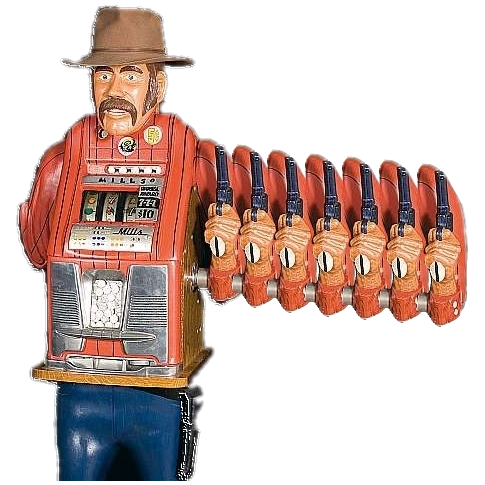# VOI-aware MCTSECAI 2012

## Multi-armed Bandits• • A set of $K$ arms.
• • Each arm can be pulled multiple times.
• • When the $i$th arm is pulled, a random reward $X_i$ is encountered.

#### Regret minimization:

• ◦ Simple regret (SR): the reward of the last pull only is collected.
• ◦ Cumulative regret (CR): all rewards are accumulated.

## UCB and UCT

• • UCB$(c)$ pulls arm $i$ that maximizes upper confidence bound $b_i$ on the reward: $b_i=\overline X_i+\sqrt {\frac {c \log (n)} {n_i}}$
• • UCB is nearly optimal in minimizing the cumulative regret.
• • UCT extends UCB to MCTS by invoking UCB at every node of a rollout.

## Metareasoning

• • A problem-solving agent can perform base-level actions from a known set $\{A_i\}$ .
• • Before committing to an action, the agent may perform a sequence of meta-level deliberation actions from a set $\{S_j\}$ .
• • At any given time there is a base-level action $A_\alpha$ that maximizes the agent's expected utility.
• • The value of information $VOI_j$ is the expected difference between the expected utilities of the new and the old selected base-level action after meta-level action $S_j$ is taken.
• • The agent selects a meta-level action that maximizes the VOI, or $A_\alpha$ if no meta-level action has positive VOI.

## Acknowledgments

• • IMG4 Consortium under the MAGNET program of the Israeli Ministry of Trade and Industry
• • Israel Science Foundation grant 305/09
• • Lynne and William Frankel Center for Computer Sciences
• • Paul Ivanier Center for Robotics Research and Production Management

## Experiments

• • VOI-based sampling is better than UCB1 for simple regret in Bandits.
• • The hybrid scheme outperforms UCT.

### Tuning the Sample Cost

Best results for sample cost $\approx 10^{-6}$:
winning rate of 64% for 10000 samples per ply.

### Winning rate vs. number of samples

#### Sample cost fixed at $10^{-6}$:

Best results for intermediate $N_{samples}$:

• • When $N_{samples}$ is too low, poor moves are selected.
• • When $N_{samples}$ is too high, the VOI of further sampling is low.

## Monte-Carlo Sampling in Trees

• • MCTS performs multiple rollouts to partially explore the search space.
• • At the current root node, the sampling is aimed at finding the first move to perform: minimizing the simple regret is more appropriate at the root node.
• • Deeper in the tree, minimizing cumulative regret results in a better estimate of the value of the state.
• • An improvement over UCT can be achieved by combining different sampling schemes on the first step and during the rest of a rollout.

## Main Results

### Hybrid sampling scheme

1. At the root node: sample based on the VOI estimate.
2. At non-root nodes: sample using UCT.

### Upper Bounds on VOI

Upper bounds on intrinsic VOI $\Lambda^b_i$ of testing the $i$th arm N times:

$$\Lambda_\alpha^b < \frac {N\overline X_\beta^{n_\beta}}{n_\alpha+1}\cdot 2\exp\left(- 1.37(\overline X_\alpha^{n_\alpha}\hspace{-0.5em} - \overline X_\beta^{n_\beta})^2 n_\alpha\right)$$ $$\Lambda_{i|i\ne\alpha}^b < \frac {N(1-\overline X_\alpha^{n_\alpha})} {n_i+1}\cdot 2\exp\left(- 1.37(\overline X_\alpha^{n_\alpha}\hspace{-0.5em} - \overline X_i^{n_i})^2 n_i\right)$$

### Sample Redistribution

MCTS re-uses rollouts generated at earlier search states.

1. Estimate VOI as though the information is discarded.
2. Stop early if the VOI is below a certain threshold.
3. Save the unused sample budget for search in future states.

The cost of a sample is the VOI of increasing a future budget by one sample.

## Contributions

• • Hybrid MCTS sampling scheme.
• • Upper bounds on VOI for simple regret in Multi-armed Bandits.
• • VOI-based stopping and sample redistribution.

## Future Work

• • Better VOI estimates.
• • VOI-based sampling for non-root nodes.
• • Application to other domains.